# Analysis of Gain and Noise in Erbium doped fiber

Compatibility:

This lesson demonstrates the performance of an amplifier (gain and noise) based on Giles and Desurvire  reference.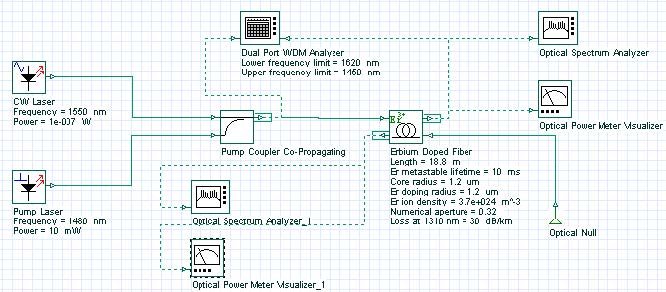Figure 1: Layout of the system considered in the analysis of gain and ASE

The characteristics of noise and gain presented in Figure 4.b  are reproduced through the system shown in Figure 1.

Figure 2 shows gain of pump and signal, and the power of backward and forward ASE as a function of the pump power. The parameters used in this simulation are displayed at Figure 1 and they are similar to the values presented in Table 1 , except for the values of the absorption and emission cross sections. Nevertheless, the results found are in good qualitative agreement with the paper.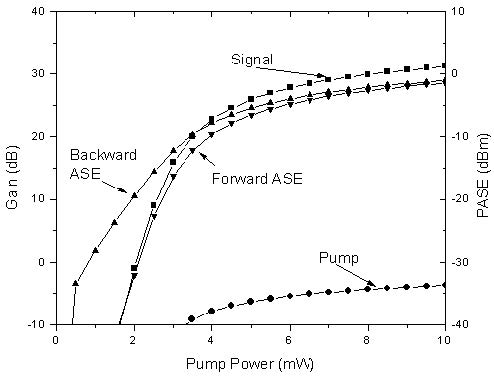Figure 2: Amplifier gain and ASE power versus pump for an Er3+-doped fiber amplifier

Note: Figure 2 equivalent to Figure 4 b) 

In this second case, the saturation effect of an amplifier is shown using two wavelength multiplexed signals at 1545 nm and 1555 nm. The system layout is shown in Figure 3 and the parameters used are similar to the parameters in Table II .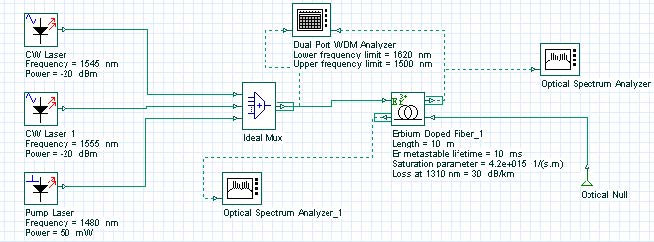Figure 3: Layout of the system used to demonstrate the saturation of the amplifier

Figure 4 shows the gain compression in the two signals due to the increase in the input power, causing the saturation of the amplifier.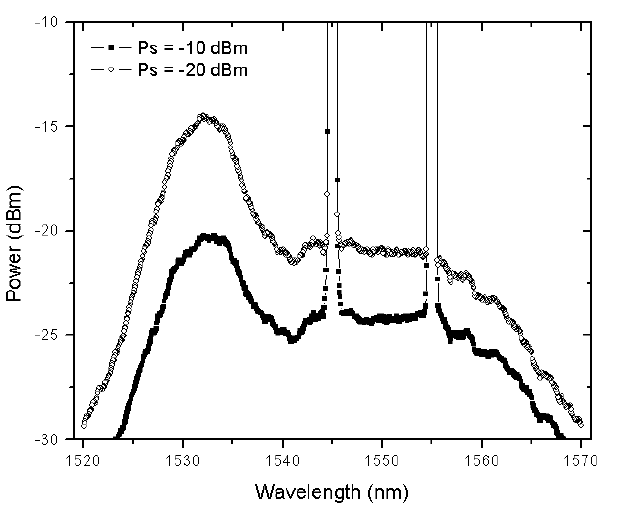Figure 4: Output spectra of a two channel amplification of a saturated amplifier

In the next case, the amplifier has two stages and a filter is inserted between the stages (see Figure 5). The effects of the equalization in the ASE are demonstrated.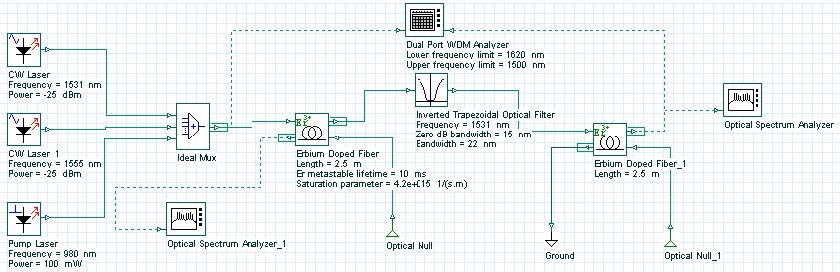Figure 5: Layout of the system used to demonstrate gain equalization in the amplifier

The EDF parameters used in this simulation are the same as in the previous case. A filter centered at 1931 nm is introduced in the middle of the EDF in a way that equalizes the gain of the two channels. Figure 6 shows the results with and without the filter. This figure is equivalent to Figure 7 in .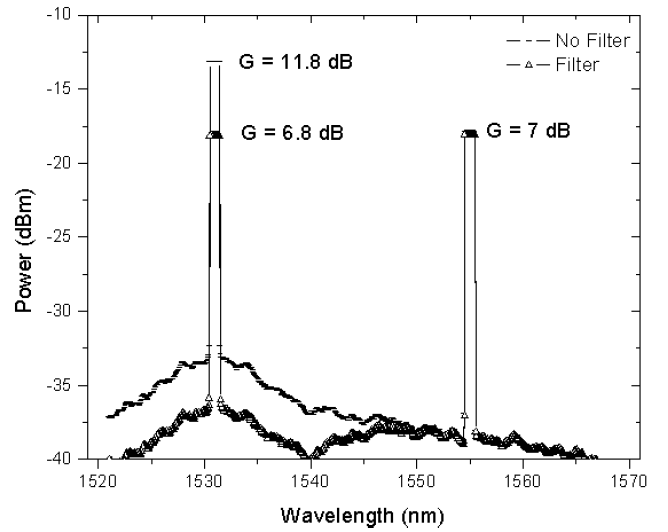Figure 6: Amplifier gain-leveling with a filter centered at 1531 nm

The results of this lesson demonstrated a qualitative agreement with the results obtained by Giles and Desurvire in , considered to be one of the most important references in the EDFA modeling.

Reference:
C. Randy Giles, and Emmanuel Desurvire, “Modeling Erbium-Doped Fiber Amplifiers”. IEEE Journal of Lightwave Technology, Volume: 9 Issue: 2, Feb. 1991, Page(s): 271 – 283.